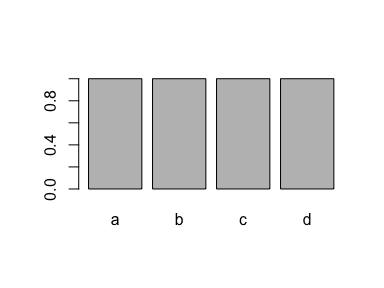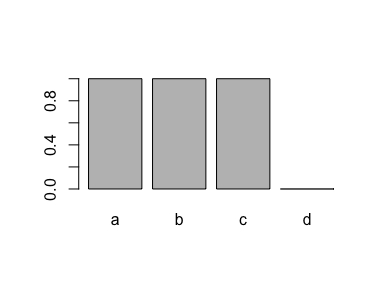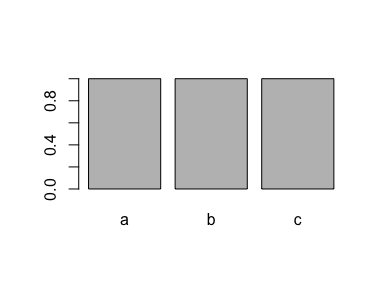# Five Tips to Improve your R Code

Five useful tips that you can use to effectively improve your R code, from using seq() to create sequences to ditching which() and much more!
Dec 2017  · 8 min read

@drsimonj here with five simple tricks I find myself sharing all the time with fellow R users to improve their code!

## 1. More Fun to Ssequence from 1

Next time you use the colon operator to create a sequence from 1 like `1:n`, try `seq()`.

``````# Sequence a vector
x <- runif(10)
seq(x)
#>    1  2  3  4  5  6  7  8  9 10

# Sequence an integer
seq(nrow(mtcars))
#>    1  2  3  4  5  6  7  8  9 10 11 12 13 14 15 16 17 18 19 20 21 22 23
#>  24 25 26 27 28 29 30 31 32
``````

The colon operator can produce unexpected results that can create all sorts of problems without you noticing! Take a look at what happens when you want to sequence the length of an empty vector:

``````# Empty vector
x <- c()

1:length(x)
#>  1 0

seq(x)
#> integer(0)
``````

You'll also notice that this saves you from using functions like `length()`. When applied to an object of a certain length, `seq()` will automatically create a sequence from 1 to the length of the object.

## 2. `vector()` what you `c()`

Next time you create an empty vector with `c()`, try to replace it with `vector("type", length)`.

``````# A numeric vector with 5 elements
vector("numeric", 5)
#>  0 0 0 0 0

# A character vector with 3 elements
vector("character", 3)
#>  "" "" ""
``````

Doing this improves memory usage and increases speed! You often know upfront what type of values will go into a vector, and how long the vector will be. Using `c()` means R has to slowly work both of these things out. So help give it a boost with `vector()`!

A good example of this value is in a for loop. People often write loops by declaring an empty vector and growing it with `c()` like this:

``````x <- c()
for (i in seq(5)) {
x <- c(x, i)
}
``````
``````#> x at step 1 : 1
#> x at step 2 : 1, 2
#> x at step 3 : 1, 2, 3
#> x at step 4 : 1, 2, 3, 4
#> x at step 5 : 1, 2, 3, 4, 5``````

Instead, pre-define the type and length with `vector()`, and reference positions by index, like this:

``````n <- 5
x <- vector("integer", n)
for (i in seq(n)) {
x[i] <- i
}
``````
``````#> x at step 1 : 1, 0, 0, 0, 0
#> x at step 2 : 1, 2, 0, 0, 0
#> x at step 3 : 1, 2, 3, 0, 0
#> x at step 4 : 1, 2, 3, 4, 0
#> x at step 5 : 1, 2, 3, 4, 5``````

Here's a quick speed comparison:

``````n <- 1e5

x_empty <- c()
system.time(for(i in seq(n)) x_empty <- c(x_empty, i))
#>    user  system elapsed
#>  15.238   2.327  17.650

x_zeros <- vector("integer", n)
system.time(for(i in seq(n)) x_zeros[i] <- i)
#>    user  system elapsed
#>   0.007   0.000   0.007
``````

That should be convincing enough!

## Master your data skills with DataCamp

More than 10 million people learn Python, R, SQL, and other tech skills using our hands-on courses crafted by industry experts.## 3. Ditch the `which()`

Next time you use `which()`, try to ditch it! People often use `which()` to get indices from some boolean condition, and then select values at those indices. This is not necessary.

Getting vector elements greater than 5:

``````x <- 3:7

# Using which (not necessary)
x[which(x > 5)]
#>  6 7

# No which
x[x > 5]
#>  6 7
``````

Or counting number of values greater than 5:

``````# Using which
length(which(x > 5))
#>  2

# Without which
sum(x > 5)
#>  2
``````

Why should you ditch `which()`? It's often unnecessary and boolean vectors are all you need.

For example, R lets you select elements flagged as `TRUE` in a boolean vector:

``````condition <- x > 5
condition
#>  FALSE FALSE FALSE  TRUE  TRUE
x[condition]
#>  6 7
``````

Also, when combined with `sum()` or `mean()`, boolean vectors can be used to get the count or proportion of values meeting a condition:

``````sum(condition)
#>  2
mean(condition)
#>  0.4
``````

`which()` tells you the indices of TRUE values:

``````which(condition)
#>  4 5
``````

And while the results are not wrong, it's just not necessary. For example, I often see people combining `which()` and `length()` to test whether any or all values are TRUE. Instead, you just need `any()` or `all()`:

``````x <- c(1, 2, 12)

# Using `which()` and `length()` to test if any values are greater than 10
if (length(which(x > 10)) > 0)
print("At least one value is greater than 10")
#>  "At least one value is greater than 10"

# Wrapping a boolean vector with `any()`
if (any(x > 10))
print("At least one value is greater than 10")
#>  "At least one value is greater than 10"

# Using `which()` and `length()` to test if all values are positive
if (length(which(x > 0)) == length(x))
print("All values are positive")
#>  "All values are positive"

# Wrapping a boolean vector with `all()`
if (all(x > 0))
print("All values are positive")
#>  "All values are positive"
``````

Oh, and it saves you a little time...

``````x <- runif(1e8)

system.time(x[which(x > .5)])
#>    user  system elapsed
#>   1.156   0.522   1.686

system.time(x[x > .5])
#>    user  system elapsed
#>   1.071   0.442   1.662
``````

## 4. `factor` that factor!

Ever removed values from a factor and found you're stuck with old levels that don't exist anymore? I see all sorts of creative ways to deal with this. The simplest solution is often just to wrap it in `factor()` again.

This example creates a factor with four levels (`"a"`, `"b"`, `"c"` and `"d"`):

``````# A factor with four levels
x <- factor(c("a", "b", "c", "d"))
x
#>  a b c d
#> Levels: a b c d

plot(x)
``````If you drop all cases of one level (`"d"`), the level is still recorded in the factor:

``````# Drop all values for one level
x <- x[x != "d"]

# But we still have this level!
x
#>  a b c
#> Levels: a b c d

plot(x)
``````A super simple method for removing it is to use `factor()` again:

``````x <- factor(x)
x
#>  a b c
#> Levels: a b c

plot(x)
``````This is typically a good solution to a problem that gets a lot of people mad. So save yourself a headache and `factor` that factor!

## 5. First you get the `\$`, then you get the power

Next time you want to extract values from a `data.frame` column where the rows meet a condition, specify the column with `\$` before the rows with `[`.

Say you want the horsepower (`hp`) for cars with 4 cylinders (`cyl`), using the `mtcars` data set. You can write either of these:

``````# rows first, column second - not ideal
mtcars[mtcars\$cyl == 4, ]\$hp
#>    93  62  95  66  52  65  97  66  91 113 109

# column first, rows second - much better
mtcars\$hp[mtcars\$cyl == 4]
#>    93  62  95  66  52  65  97  66  91 113 109
``````

The tip here is to use the second approach.

But why is that?

First reason: do away with that pesky comma! When you specify rows before the column, you need to remember the comma: `mtcars[mtcars\$cyl == 4`,`]\$hp`. When you specify column first, this means that you're now referring to a vector, and don't need the comma!

Second reason: speed! Let's test it out on a larger data frame:

``````# Simulate a data frame...
n <- 1e7
d <- data.frame(
a = seq(n),
b = runif(n)
)

# rows first, column second - not ideal
system.time(d[d\$b > .5, ]\$a)
#>    user  system elapsed
#>   0.497   0.126   0.629

# column first, rows second - much better
system.time(d\$a[d\$b > .5])
#>    user  system elapsed
#>   0.089   0.017   0.107
``````

Worth it, right?

Still, if you want to hone your skills as an R data frame ninja, I suggest learning `dplyr`. You can get a good overview on the `dplyr` website or really learn the ropes with online courses like DataCamp's Data Manipulation in R with `dplyr`.

## Sign off

Thanks for reading and I hope this was useful for you.

For updates of recent blog posts, follow @drsimonj on Twitter, or email me at [email protected] to get in touch.

If you'd like the code that produced this blog, check out the blogR GitHub repository.

Check out our Getting Started with the Tidyverse: Tutorial.

R Courses

### .css-1531qan{-webkit-text-decoration:none;text-decoration:none;color:inherit;}Introduction to R

Beginner
4 hr
2.5M
Master the basics of data analysis in R, including vectors, lists, and data frames, and practice R with real data sets.
See Details

### Intermediate R

Beginner
6 hr
555.1K
Continue your journey to becoming an R ninja by learning about conditional statements, loops, and vector functions.

### Introduction to the Tidyverse

Beginner
4 hr
280.2K
Get started on the path to exploring and visualizing your own data with the tidyverse, a powerful and popular collection of data science tools within R.
See More
Related

### Building Your Data Science Portfolio with DataCamp Workspace (Part 1)

Learn how to build a comprehensive data science portfolio by exploring examples different examples, mastering tips to make your work stand out, and utilizing the DataCamp Workspace effectively to showcase your results.9 min### [Radar Recap] Building an Enterprise Data Strategy that Puts People First

Cindi Howson and Valerie Logan discuss how data leaders can create a data strategy that puts their people at the center.### [Radar Recap] Unleashing the Power of Data Teams in 2023

Vijay Yadav and Vanessa Gonzalez will outline the keys to building high-impact data teams in 2023.### The Past, Present, and Future, of the Data Science Notebook

Jodie Burchell discusses notebooks and the challenges facing data science today.### Building a Safer Internet with Data Science

Learn the key drivers of a data strategy that helps ensure online safety and consumer protection with Richard Davis, the Chief Data Officer at Ofcom, the UK’s government-approved regulatory and competition authority.

### Conda Cheat Sheet

In this cheat sheet, learn all about the basics of working with Conda. From managing and installing packages, to working with channels & environments, learn the fundamentals of the conda package management tool suite.
See MoreSee More